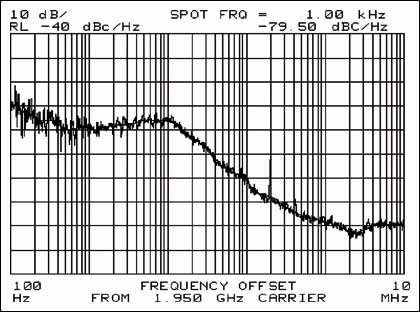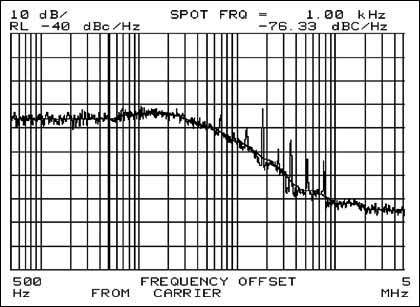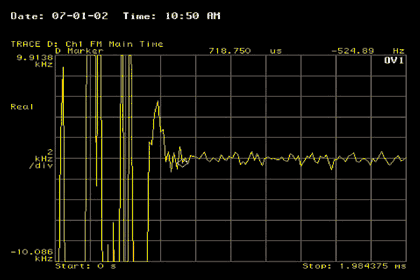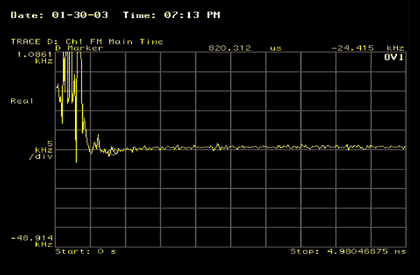# Performance of the MAX2395 PLL with 80kHz Comparison Frequency

### Abstract

This application note presents an optimized loop filter design for the MAX2395 with 80kHz compare frequency to support WCDMA/UMTS systems. The reference frequency is 13MHz or 26MHz. EVM results are also shown.

### Introduction

The MAX2395 is a fully monolithic quasi-direct conversion modulator IC made for use in WCDMA/UMTS transmitters. Due to its internal architecture, the RF output frequency is at 5/6 of the RF LO frequency, which is generated from an on-chip integer-N PLL. Since the raster frequency in a WCDMA system is 200kHz, the MAX2395 PLL needs to provide a 240kHz step size (i.e., 6/5 times 200). For the most popular reference frequencies in WCDMA user-terminal applications, such as 19.2MHz and 15.36MHz, the 240kHz comparison frequency can be easily generated by the internal PLL integer divider, 80 and 64 respectively. The Maxim evaluation board was designed with a 19.2MHz reference in mind.

In a UMTS system, a 13MHz or 26MHz reference frequency is often used, which is not an integer multiple of 240kHz. So the comparison frequency used by the MAX2395's PLL needs to be reduced to a number whose multiple is 240kHz and 13/26MHz. For the 26MHz crystal, the comparison frequency commonly used is 80kHz, which permits a 240kHz channel raster by using three 80kHz steps. The 80kHz PLL frequency represents 3 times greater "divide-by-N" value for the same LO, which results in 10dB increased close-in phase noise (20logN).

The EVM (error vector magnitude) will be also degraded, since the PLL contribution to EVM is roughly proportional to its integrated phase error. The comparison spurs get lower attenuation from the loop filter, so the loop filter needs to be re-designed to balance integrated phase error, settling time and comparison spurs attenuation.

### Loop Filter Components

The loop filter bandwidth used at 240kHz comparison frequency is around 12kHz, and is approximately 7kHz bandwidth for the 80kHz comparison frequency case. The corresponding loop filter component values are shown in the following table:

Table 1.

 BW = 12kHz, 240kHz comparison freq. BW = 7kHz, 80kHz comparison freq. C1 = 2.2nF C1 = 1nF C2 = 22nF C2 = 10nF R2 = 3.3kΩ R2 = 7.5kΩ

See the schematic below, (Figure 1) for the passive loop-filter topology.Figure 1.

### Measured Data

The MAX2395 performance was measured on the Maxim WCDMA reference design board using 19.2MHz and 26MHz crystals respectively. All the measured plots are presented in Figures 2 to 7.Figure 2. Phase noise plot at 19.2MHz reference crystal.Figure 3. Phase noise plot at 26MHz reference crystal.Figure 4. EVM at RF output at 19.2MHz reference crystal.Figure 5. EVM at RF output at 26MHz reference crystal.Figure 6. PLL Settling time over 60MHz jump at 19.2MHz crystal.Figure 7. PLL settling time over 60MHz jump at 26MHz crystal.

The integrated phase error from 500Hz to 1.92MHz is 1.98 degrees at 240kHz comparison frequency and 2.75 degrees at 80kHz comparison frequency. The former has EVM results at the RF output of 5.5%, while the latter yields 7.2% EVM. The loop settling time is 720µs for a 60MHz step frequency using a 19.2MHz crystal, and 820µs settling time for the 26MHz crystal. The comparison spur attenuation is similar in both cases, around -40dBc.